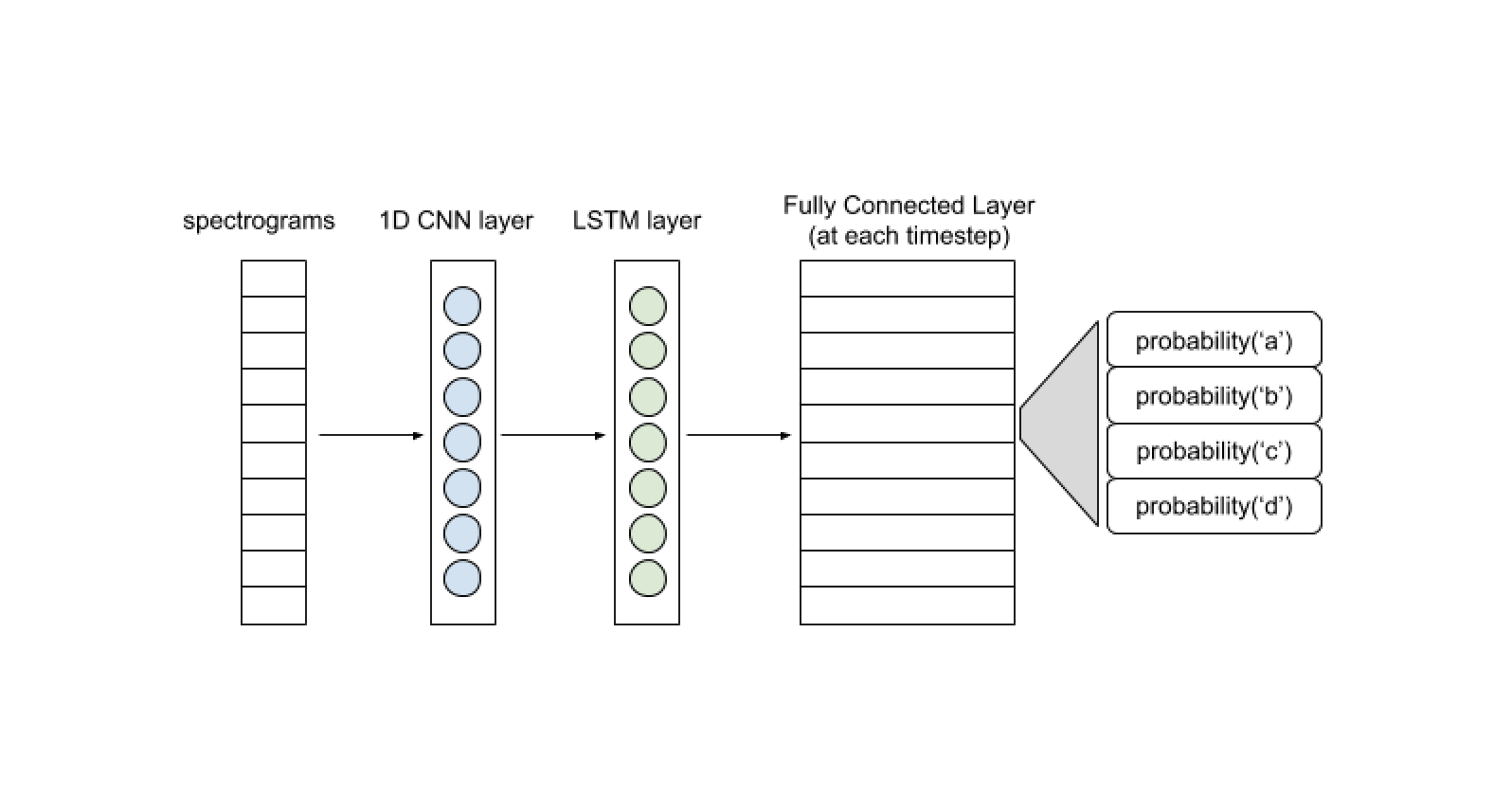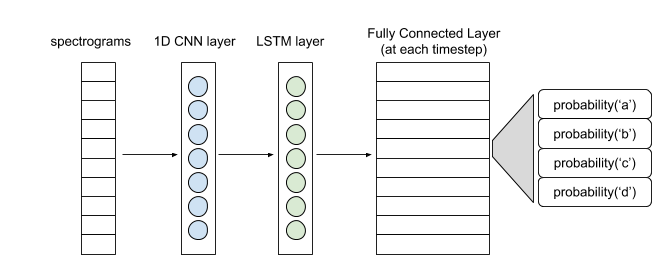📈📈📈 G2 Momentum Continues for Observe.AI 📈📈📈 Read the Blog ---># Hello World in Speech Recognition

Stripped down end to end ASR in Tensorflow. This blog will help you write a basic end to end ASR system using Tensorflow.

This blog walks through writing a basic end-to-end Automatic Speech Recognition (ASR) system using Tensorflow. I will go over each component of a minimal neural network and a prefix beam search decoder required to generate a readable transcript from audio.

I've come across a lot of resources on building basic machine learning systems around computer vision and natural language processing tasks, but very few when it comes to speech recognition. This is an attempt to fill that gap and make this field less daunting for beginners.

## Prerequisites

Familiarity with:

• Components of a neural network
• Training a neural network
• Using a language model to get the probability of a sequence of words

## Overview

• Audio preprocessing: Converting raw audio into numerical features which are useful as inputs for a neural network.
• Neural Network: A simple architecture for converting audio features into probability distributions over the possible characters in the transcript.
• CTC loss: Calculating the loss without annotating each timestep of the audio with its corresponding character.
• Decoding: Creating a transcript from the probability distributions for each timestep using prefix beam search and a language model.

I will be focusing on the Neural Network, CTC loss, and Decoding part.

## Audio Preprocessing

You need to convert your audio into a feature matrix to feed it into your neural network. One simple way is to create spectrograms.

Code is available here.

This function computes the Short-time Fourier Transform of your audio signal and then computes the power spectrum. The output is a matrix called spectrogram. You can directly use this as your input. Other alternatives to this are filter banks and MFCCs. Audio preprocessing is a whole topic in itself. You can read about it in detail here.

## Neural Network

Here is a simple architecture.Hello World Architecture for Speech Recognition

The spectrogram input can be thought of as a vector at each timestamp. A 1D convolutional layer extracts features out of each of these vectors to give you a sequence of feature vectors for the LSTM layer to process. The output of the (Bi)LSTM layer is passed to a Fully Connected layer for each time step which gives a probability distribution of the character at that time step using softmax activation. This network will be trained with CTC (Connectionist Temporal Classification) loss function. Feel free to experiment with more complex models after understanding the entire pipeline.

Code is available here.

### Why CTC?

This network attempts to predict the character at each timestep. Our labels, however, are not the characters at each timestep but just the transcription of the audio. Keep in mind that each character in the transcription may stretch across multiple timesteps. The word C-A-T will come across as C-C-C-A-A-T-T if you somehow label each timestep in the audio.

Annotating your audio dataset at every 10ms is not feasible. CTC solves this problem as it does not require us to label every timestep. It takes as input the entire output probability matrix of the above neural network and the corresponding text, ignoring the position and actual offsets of each character in the transcript.

## CTC Loss Calculation

Suppose the ground truth label is CAT. Within these four timesteps, sequences like C-C-A-T, C-A-A-T, C-A-T-T, _-C-A-T, C-A-T-_ all correspond to our ground truth. We will calculate the probability of our ground truth by summing up the probabilities for all these sequences. The probability of a single sequence is calculated by multiplying the probabilities of its characters as per the output probability matrix.

For the above sequences, the total probability comes out to be 0.0288 + 0.0144 + 0.0036 + 0.0576 + 0.0012 = 0.1056. The loss is the negative logarithm of this probability. The loss function is already implemented in TensorFlow. You can read the docs here.

## Decoding

The output you get from the above neural network is the CTC matrix. CTC matrix gives the probability of each character in its set at each timestep. We use Prefix Beam Search to make meaningful text out of this matrix.

The set of characters in the CTC matrix has two special tokens apart from the alphabet and space character. These are blank token and end of string token.

Purpose of blank token: The timestep in the CTC matrix is usually small. (~10 ms) So each character of the spoken sentence stretches across multiple timesteps. For example, C-A-T becomes C-C-C-A-A-T-T. Therefore we collapse all repetition across the possible candidate strings that stand out in the CTC matrix. What about words like FUNNY where N is supposed to repeat? A blank token between the two Ns prevents them from collapsing into one without adding anything in the text. So, F-F-U-N-[blank]-N-N--Y collapses into FUNNY.

Purpose of end-token: End-of-string denotes the end of the spoken sentence. Decoding at timesteps after end-of-string token does not add anything to the candidate string.

## Procedure

Initialization:

• We have a list of candidates initially. It consists of a single blank string. The list also contains the probability of that candidate ending in a blank token and ending in a non-blank token at each timestep. The probability of the blank string ending in a blank token at time 0 is 1. The probability of it ending in a non-blank token is 0.

Iterations:

• We take this string and add each character to it one by one. We take each extended string formed and calculate its probability of ending in a blank and non-blank token at time=1. Then we store these extended strings along with their probabilities on our list. We put these new candidates on our list and repeat the process for the next timestep.
• Case A: If the added character is a blank token, we make no change in the candidate.
• Case B: If the added character is a space, we multiply the probability with a number proportional to the probability of the candidate as per a language model. This prevents incorrect spellings from becoming the best candidate. So COOL will not be spelled as KUL in the final output.
• Case C: If the added character is the same as the last character of the candidate. (candidate=FUN. character=N) We create two new candidates, FUNN and FUN. The probability of FUN is calculated from the probability of FUN ending in a blank token. The probability of FUNN is calculated using the probability of FUN ending in a non-blank token. So, if FUN does not end with the blank token, we discard the additional N instead of appending it.

Output:
The best candidate after all the timesteps is the output.

We make two modifications to make this process faster.

1. After each timestep, we discard all but the best K candidates. The candidates are sorted by the sum of their probability of ending in a blank and non-blank token.
2. We do not consider the characters which have their probability in the matrix below a certain threshold (~0.001).

Go through the code below for implementation details.

Code is available here.

This completes a bare-bones speech recognition system. You can introduce a bunch of complications to get better outputs. Bigger networks and audio preprocessing tricks help a lot. Here is the complete code.

Notes:
1. The code above uses TensorFlow 2.0 and the sample audio file has been taken from the LibriSpeech dataset.
2. You will need to write your own batch generators to train over an audio dataset. These implementation details are not included in the code.
3. You will need to write your own language model function for the decoding part. One of the simplest implementations would be to create a dictionary of bigrams and their probabilities based on some text corpus.

References:
 A.Y. Hannun et al., Prefix Search Decoding (2014), arXiv preprint arXiv:1408.2873, 2014
 A. Graves et al., CTC Loss (2006), ICML 2006
 L. Borgholt, Prefix Beam Search (2018), Medium

No items found.
Want more like this straight to your inbox?
Subscribe to our newsletter.
Thanks for subscribing. We've sent a confirmation email to your inbox.
Oops! Something went wrong while submitting the form.
SCHEDULE A DEMO

## Deliver breakthrough results with the Intelligent Workforce Platform